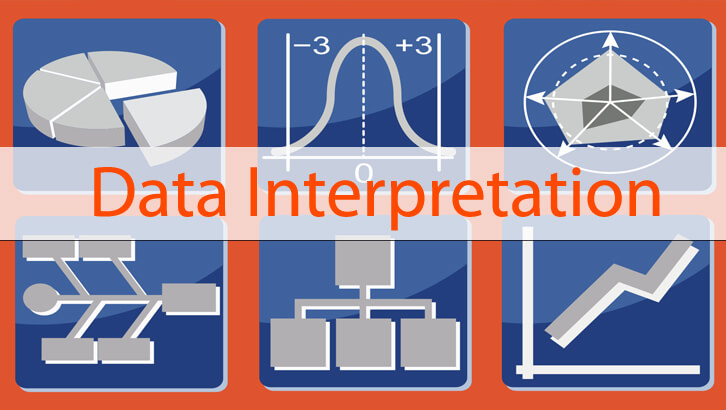# Data Interpretation – Average growth rate vs. CAGR

Growth rate, average growth rate, CAGR – these are three terms that appear frequently in exams. I am going to examine each of this with an example.

Let us say company XYZ has revenues in 5 consecutive years as follows

2011 –  Rs. 1200 crores
2012 –  Rs. 1250 crores
2013 –  Rs. 1310 crores
2014 –  Rs. 1380 crores
2015 –  Rs. 1500 crores

For the period from 2011 to 2015, we can calculate all three – growth rate, average annual growth and CAGR. Let us do each of this.

Growth rate from 2011 to 2015.
Revenues have grown from Rs. 1200 crores to Rs. 1500 crores, a growth of Rs. 300 crores. Growth rate = 300/1200 expressed as a percentage = 25%.

Average annual growth rate from 2011 to 2015
We need to calculate growth rate in each year and then compute the average of those growth rates

Year     Revenues                            growth rate

2011 –  Rs. 1200 crores
2012 –  Rs. 1250 crores                        4.2%
2013 –  Rs. 1310 crores                        4.8%
2014 –  Rs. 1380 crores                        5.3%
2015 –  Rs. 1500 crores                        8.7%

Average of 4.2%, 4.8%, 5.3% and 8.7% = 5.75%

CAGR of revenues from 2011 to 2015
The CAGR is an interesting idea. Conceptually, it is that equal growth rate, if it had been present for all 4 years that would have taken us from Rs. 1200 crores to 1500 crores. Or, if we assume some growth rate ‘r’ in each year that takes us from the starting point to the end point, that r is the CAGR.

So, in our question 1200( 1 + r)^4 = 1500.

How so, each year’s revenues would be (1 + r) times the previous year’s revenue. So, the final year’s revenue would be (1 + r) ^4 of the first year’s revenue.

(1 + r)^4 = 1.25

Or, CAGR = 5.73%.

Usually, CAGR and average growth rate will be reasonably close to each other. Both give a reasonable sense of how things have been. If the growth rates are wildly variant, then CAGR and average growth rate will be far apart. Otherwise they will be close to each other.

Why CAGR is often better
Imagine a stock price that jumps by 100% in year 1 and falls by 50% in the second year. Growth rates are 100% and -50%. Average growth rate would be 25%. Sounds like fantastic returns for an investor. But this is wrong. Dramatically wrong. If the stock’s original price had been Rs. 20. At the end of year 1, it would have been Rs. 40, at the end of year 2 it would have been back to Rs. 20. So, the overall growth is zero. Zlich. Nada. CAGR is 0. Remember that percentages are calculated on the starting point. So, for the same change, going up will you will see a higher percentage growth than coming down. If a stock goes from 100 to Rs. 1000 it is a 900% growth rate. If it goes the other way around, it is only a 90% decline. Oops!

So, if any mutual fund manager pitches his fund to you based on average growth, ask him for the CAGR and then politely ask him to leave. 🙂

How to compute CAGR using a calculator.
Let us do this with an example. If revenues from \$300m to \$ 369m in 4 years, what is the CAGR? 300( 1+r)^4 = 369.

(1+r)^4 = 369/300 = 1.23
(1+r) = 1.23^0.25 = 1.053.
r = 5.3%

If we go from Rs. x to Rs. y in ‘n’ years. x(1+r)^n = y.
(1 + r) = (y/x) ^ (1/n)

r = (y/x)^(1/n) – 1.

Now, remember, this formula will give ‘r’ as 0.625 or something like that. We need to think of it as 6.25%.

Let us finish with another example.
Revenues grow from Rs. 6000 crores to Rs. 8250 crores in 5 years, what is the CAGR?
8250/6000 = 1.375
1.375^0.2 = 1.06576.
Or, r = 0.06576 or 6.58%.